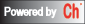# CGI-based Web Numerical Calculation of Integration of Ordinary Differential Equations

## Integrate with Single ODE function

```
X-min:                 X-max:
dy/dx =  Initial Value y =

What outputs do you want to display:

Function chart
Data table

```

## Integrate with Two sets of ODE functions

```
X-min:                     X-max:
dy/dx =  Initial Value y =
dy/dx =  Initial Value y =

What outputs do you want to display:

Function chart
Data table

```

## Integrate with more than Two sets of ODE functions

Number of differential equations :